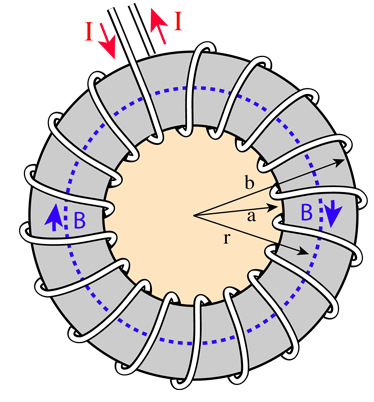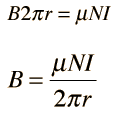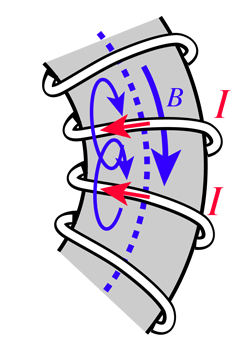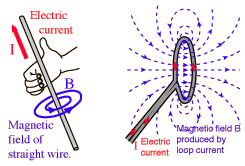# Magnetic Field of ToroidFinding the magnetic field inside a toroid is a good example of the power of Ampere's law. The current enclosed by the dashed line is just the number of loops times the current in each loop. Amperes law then gives the magnetic field byThe toroid is a useful device used in everything from tape heads to tokamaks.
 Toroid section detail Magnetic field calculation
Index

Magnetic field concepts

 HyperPhysics***** Electricity and Magnetism R Nave
Go Back

# Magnetic Field of ToroidFinding the magnetic field inside a toroid is a good example of the power of Ampere's law. The current enclosed by the dashed line is just the number of loops times the current in each loop. Amperes law then gives the magnetic field byThe toroid is a useful device used in everything from tape heads to tokamaks.
Magnetic field = permeability x turn density x current

For a solenoid of radius r = m with N = turns,
the turn density is n=N/(2πr)= turns/m.

If the current in the solenoid is I = amperes

and the relative permeability of the core is k = ,

then the magnetic field at the center of the solenoid is

B = Tesla = gauss.

The Earth's magnetic field is about half a gauss.

The relative permeability of magnetic iron is around 200.

Enter data, then click on the quantity you wish to calculate in the active formula above the illustration. Default values will be entered for unspecified parameters, but the numbers will not be forced to be consistent until you click on the quantity to calculate.

 Toroid section detail Toroid magnetic field
Index

Magnetic field concepts

 HyperPhysics***** Electricity and Magnetism R Nave
Go Back

# Toroid DetailAll of the loops of wire which make up a toroid contribute magnetic field in the same direction inside the toroid. The sense of the magnetic field is that given by the right hand rule, and a more detailed visualization of the field of each loop can be obtained by examining the field of a single current loop.Toroid magnetic field Field calculation
Index

Magnetic field concepts

 HyperPhysics***** Electricity and Magnetism R Nave
Go Back